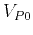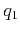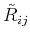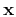On anelliptic approximations for qP velocities in TI and orthorhombic mediaNext: Bibliography Up: Sripanich & Fomel: qP Previous: Acknowledgments

# Appendix A: Uncertainty Analysis

In this appendix, we study the variation of the phase-velocity expression in TI media with respect to different choices of parameters, particularly Thomsen's parameters and the proposed Muir-Dellinger parameters. To study resolution, we use the general formula,(65)

whereis the exact phase-velocity expression (equation 9),andare two of the four parameters present in the expression, andis the phase angle measured from vertical. The matrix, in both cases, is computed based on the stiffness tensor of Greenhorn shales given in Table 6. The results are shown in Tables 10 and 11. Note that the matrix is symmetric, so the values are shown only on one side of the diagonal.

 Parameters87.11 0.467 105.39 25.190.005 0.649 0.20181.74 22.5413.16

Table 10.of the exact phase-velocity expression with the two considered Thomsen parameters are denoted in each row and column.

 Parameters0.576 0.143 0.651 0.5990.538 0.257 1.0611.325 1.2794.124

Table 11.of the exact phase-velocity expression with the two considered anelliptic parameters are denoted in each row and column.

Table 10 shows a significantly larger correlation between the change in phase velocity within comparison with that of, which agrees with the general assumption of the independency ofin qP velocities approximations. Likewise, the effect fromhas a higher correlation with the change of phase velocity thanbecause the exact qP phase-velocity formula (equation 9) can be expressed in terms of Thomsen parameters withcorresponding to the lower order ofthan. Moreover,andalso have high correlation with, which is apparent from their definitions.

Table 11 shows relatively similar correlations fromandto the change in exact phase velocity suggesting a more symmetric contribution from both parameters. The dimensionless anelliptic parametersandexhibit a strong correlation, which is consistent with the relationships shown in Figure 1.

By ignoring the effect ofin the case of Thomsen parameters or using the relationship betweenand(Figure 1) to reduce the number of parameters to three, we can transform the matrixfromto(). Note that the matrix for Thomsen parameters is similar to Table 10 with the omittance of the row and column associated with. Table 12 shows the three-parameter matrix for anelliptic parameters with similar behavior of relatively equal correlations fromandas before.

 Parameters0.578 0.144 1.1660.534 1.2867.411

Table 12.of the exact phase-velocity expression with the two considered anelliptic parameters are denoted in each row and column.

To better visualize the variational effect from the change in the three parameters in both cases, we follow the approach of Osypov et al. (2008), compute the quadratic form ofand plot its contour at a given amount of change in the exact phase velocity expression,(66)

wheredenotes the vector of parameter variations: [,,]or [,,]andis computed at the known values of the anisotropic parameters of the model (Greenhorn shales). The resultant plots are shown in Figure A-1. For Thomsen's parameters, Figure A-1a shows a strongly oblate ellipsoid with high degree of deviation (stretch) from a sphere for all three parameters. On the contrary, Figure A-1b shows oblate ellipsoid with smaller deviation suggesting that the Muir-Dellinger parameters may represent a more orthogonal parameterization scheme than Thomsen's parameters. This observation is important for the problem of estimating anisotropic parameters, which goes beyond the scope of this paper.thomsenmatrix,zonematrix
Figure 14.
Ellipsoids obtained from the quadratic form ofin the case of a) Thomsen parameters b) anelliptic parameters.On anelliptic approximations for qP velocities in TI and orthorhombic mediaNext: Bibliography Up: Sripanich & Fomel: qP Previous: Acknowledgments

2017-04-14